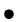Next:9.11 To Probe FurtherUp:9. Sorting MethodsPrevious:9.9 Radix Sorting

# 9.10 Merge Sort

• Divide and conquer algorithm
• O(nlog n) worst case performance
• Useful in external sorting applications.
Algorithm

list-type mergesort (list-type L; int n)

{

if (n = 1)

return (L)

else {

split L into two halves L1and L2;

return (merge (mergesort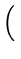L1,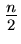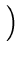, mergesort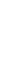L2,}

}

Let T(n) be the running time of mergesort on a list of size n. Then.

 T(n)C1   (n = 1)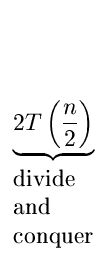+(n > 1)

It can be shown that T(n) is O(nlog n).

Mergesort as an External Sorting Procedure

• Assume that the data to be sorted is stored in a file
• The essential idea is to organize a file into progressively larger runs where:

•

A run is a sequence of records r1,..., rk, such that ri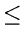ri + 1 for i = 1,..., k - 1.

• We say a file, r1, r2,..., rm, of records is organized into runs or length k if: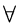i1 such that kim, the sequence
•rk(i - 1) + 1, rk(i - 1) + 2,..., rkiis a run of length k and furthermore if m is not divisible by k and m = pk + q where q < k, the sequence (rm - q + 1,..., rm) which is called the tail is a run of length q.
Example

The following is organized into runs of length 3 with the tail having length 2.

 7 15 29 8 11 13 16 22 31 5 12
Basic step

Consider two files f1 and f2 organized into runs of length k. Assume that

1.
If ni is the number of runs (including tails) of f1(i = 1, 2), then | n1 - n21
2.
At most one of f1 and f2 has a tail
3.
The one with a tail (if any) has at least as many runs as the other.
In mergesort, we read one run from each of f1 and f2, merge the runs into a run of length 2k and append it to one of two files g1 and g2. By alternating between g1 and g2, these files would be organized into runs of length 2k, satisfying (1), (2), and (3).

Algorithm

• First divide all n records into two files f1 and f2 as evenly as possible. f1 and f2 can be regarded as organized into runs of length 1.
• Merge the runs of length 1 and distribute them into files g1 and g2which will now be organized into runs of length 2.
• Now make f1 and f2 empty and merge g1 and g2 into f1 and f2 creating runs of length 4.
Repeat ...

Example

 f1 28 3 93 54 65 30 90 10 69 8 22 f2 31 5 96 85 9 39 13 8 77 10
runs of length 1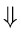merge into g1 and g2

 g1 28 31 93 96 9 65 13 90 69 77 22 g2 3 5 54 85 30 39 8 10 8 10
runs of length 2merge into f1 and f2

 f1 3 5 28 31 9 30 39 65 8 10 69 77 f2 54 85 93 96 8 10 13 90 22
runs of length 4merge into g1 and g2

 g1 3 5 28 31 54 85 93 96 8 10 22 69 77 g2 8 9 10 13 30 39 65 90
runs of length 8merge into f1 and f2

 f1 3 5 8 9 10 13 28 30 31 39 54 65 85 90 93 96 f2 8 10 22 69 77
runs of length 16merge into g1 and g2

g1 will be left with the sorted sequence

• After i passes, we have two files consisting of runs of length 2i. If 2i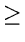n, then one of the two files will be empty and the other will contain a single run of length n (which is the sorted sequence).

•

Therefore number of passes =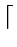logn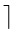• Each pass requires the reading of two files and the writing of two files, all of length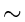.

•

Total number of blocks read or written in a pass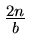(where b is the number of records that fit into a block)

Total number of block reads or writes for the entire sorting}O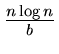.

• The procedure to merge reads and writes only one record at a time. Thus it is not required to have a complete run in memory at any time.
• Mergesort need not start with runs of length 1. We could start with a pass that, for some appropriate k, reads groups of k records into main memory, sorts them (say quicksort) and writes them out as a run of length k.
Multiway Mergesort
• Here we merge m files at a time. Let the files f1,..., fm be organized as runs of length k.

•

We can read m runs, one from each file, and merge them into one run of length mk. This run is placed on one of m files g1,..., gm, each getting a run in turn.

• The merging process will involve each time selecting the minimum among m currently smallest unselected records from each file.

•

By using a priority queue that supports insert and delete in logarithmic time (e.g., partially ordered tree), the process can be accomplished in O(log m)time.

• Number of passes -logmn• Effort in each pass = O(nlog2m)
overall complexityO(nlog2m.logmn)
•